# Apply IC in Cryptocurrency Exchange || Be a rational trader

in hive-167922 •  11 days ago

# Indifference Curve in Cryptocurrencies

An indifference curve is a geometrical presentation of a consumer in scale of preferences. It represents all combinations of two goods which will provide equal satisfaction to a consumer..

As a trader of different currencies, you may consider your indifference curve to compare about different Cryptocurrencies and NFTs. It will give you comparative idea about different currencies and self-analysis to take proper decision about trading.

A consumer is indifferent towards the different combinations located on such a curve. Since each combination yields the same level of satisfaction, the total satisfaction derived from any of these combinations remains constant.

Suppose the following schedule indicates different combinations of BTC & ETH namely A, B, C and D yield equal satisfaction to a Cryptocurrency Trader.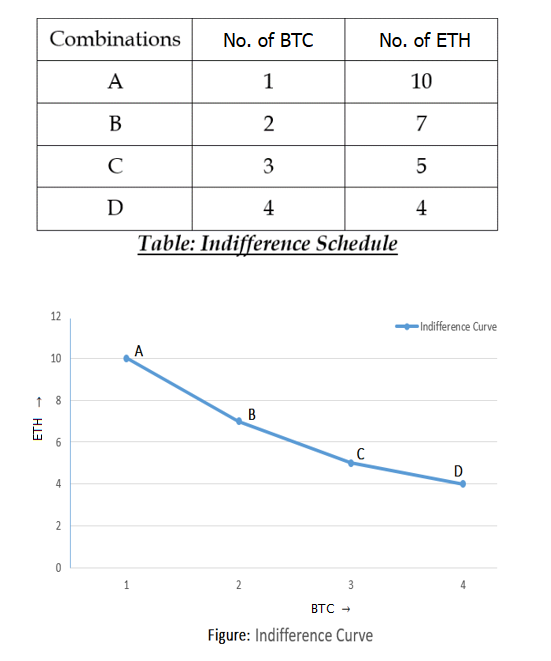In combination A the consumer has 1 BTC & 10 ETHs, in combination B he has 2 BTCs & 7 ETHs, in combination C he has 3 BTCs & 5 ETHs, and in combination D he has 4 BTCs & 4 ETHs.

In order to have one more BTC the consumer sacrifice, some of the ETHs in such a way that there is no change in the level of his satisfaction out of, each combination.

Diagrammatic representation of indifference schedule is Indifference curve. In this diagram, quantity of BTCs is shown on X-axis & ETHs on Y-axis. AD is an indifference curve.

Different points A, B, C & D on it indicate those combinations of BTCs and ETHs which yield equal satisfaction to the consumer.

# Properties of Indifference Curves:

Now we will explain the properties and features of the indifference Curve in case of Cryptocurrencies as we can conduct it with our daily transactions..

### 1. Indifference curve slopes downward/negative slope:

The downward slope of an indifference curve indicates that a consumer will have to curtail the consumption of one commodity if he wants to consume large quantity of another commodity to maintain the same level of satisfaction.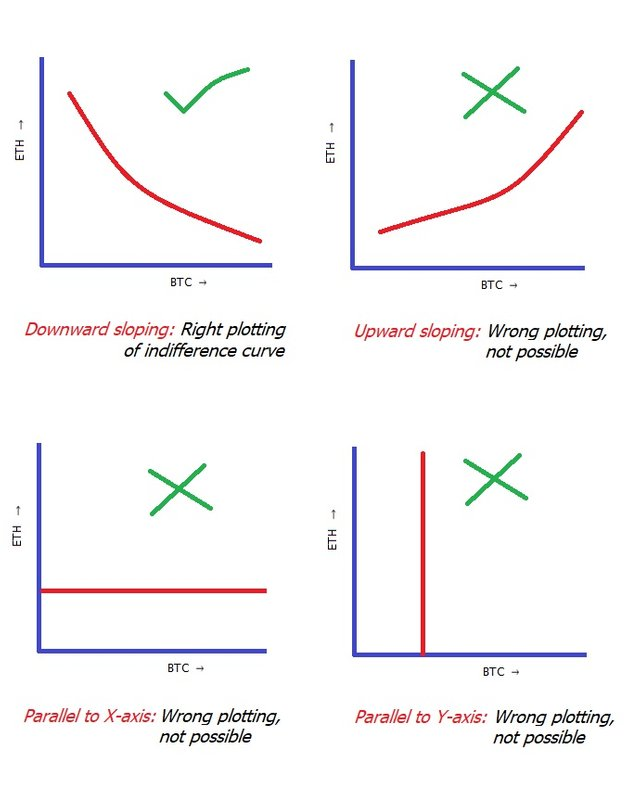An indifference curve cannot be upward sloping or parallel to X-axis or parallel to Y-axis

### 2. Indifference curve is convex to the point of origin:

An indifference curve is convex to the point of origin. This property is based on the law of diminishing marginal rate of substitution.

A downward sloping straight line indifference curve can be possible only in case of perfect substitutes which is not possible in real life. Because any two goods cannot be perfect substitutes.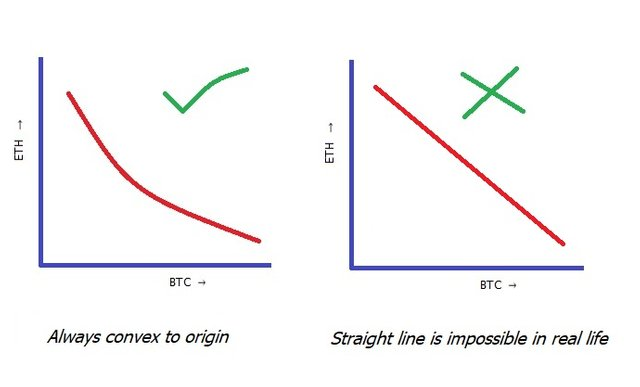As a trader of BTC & ETH, you must have some extra satisfaction to either BTC, or ETH. As the currencies cannot be perfect substitutes to each other, so a downward sloping straight line indifference curve isn’t possible.

### 3. Two Indifference Curves never cut each other: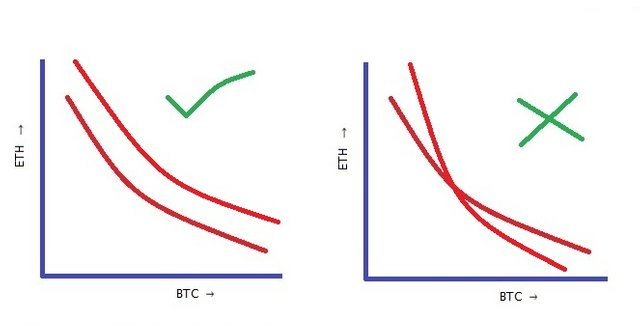Each indifference curve represents different levels of satisfaction, so their intersection is ruled out.

### 4. Higher Indifference Curve represent more satisfaction: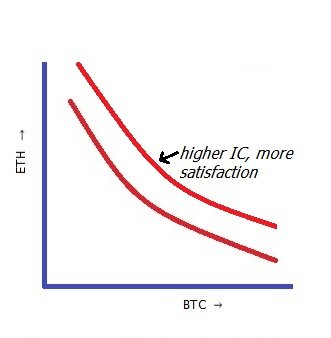In a diagram if any two indifference curve where one is higher than another, it signifies that higher the indifference curve, greater the satisfaction it represents.

### 5. Indifference Curve touches neither x-axis nor y-axis:

In case an indifference curve touches either axis it means that the consumer wants only one commodity and his demand for the second commodity is zero.

An indifference curve may touch Y-axis if it represents money instead of a commodity.

### 6. Indifference curves need not be parallel to each other:

Indifference curves may or may not be parallel to each other. It all depends on the marginal rate of substitution on two curves shown in the indifference map.

If marginal rate of substitution of different points on two curves diminishes at constant rate, then these curves will be parallel to each other, otherwise they will not be parallel.

### 7. Indifference curves become complex in case of more than two commodities:

When a consumer desires to have combinations of more than two commodities, say, three commodities like BTC, ETH & HBD, we will have to draw three dimensional indifference curves which are quite complex.If the consumer wants a combination comprising of more than three goods, such a combination cannot be expressed in the form of a diagram.##### My Footsteps on Virtual World:

"I am an engineer by graduation
a teacher by occupation
& a writer by passion"

``````        I believe, Life is beautiful!
``````

Posted Using LeoFinance